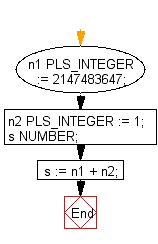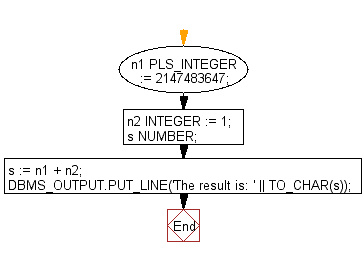﻿ PL/SQL DataType Exercises: Program to show the upper limit of PLS_INTEGER - w3resource# PL/SQL DataType: Program to show the upper limit of PLS_INTEGER

## PL/SQL DataType: Exercise-5 with Solution

Write a PL/SQL program to show the upper limit of PLS_INTEGER.

Sample Solution:

PL/SQL Code:

``````DECLARE
n1 PLS_INTEGER := 2147483647;
n2 PLS_INTEGER := 1;
s NUMBER;
BEGIN
s := n1 + n2;
END;
/
```
```

Sample Output:

```DECLARE
*
ERROR at line 1:
ORA-01426: numeric overflow
ORA-06512: at line 6
```

Flowchart:The range of the PLS_INTEGER datatype is -2,147,483,648 through 2,147,483,647. In the expression s:= n1 + n2, exceded the upper limit.

A calculation with two PLS_INTEGER values overflows the PLS_INTEGER range and an overflow exception error message will appear, even if the result assign to a NUMBER data type.

To prevent the exception overflow use integer for calculations outside the PLS_INTEGER range. Here is the example:

``````DECLARE
n1 PLS_INTEGER := 2147483647;
n2 INTEGER := 1;
s NUMBER;
BEGIN
s := n1 + n2;
DBMS_OUTPUT.PUT_LINE('The result is: ' || TO_CHAR(s));
END;
/
```
```

Sample Output:

```The result is: 2147483648

PL/SQL procedure successfully completed.
```

Flowchart:Improve this sample solution and post your code through Disqus

What is the difficulty level of this exercise?

﻿